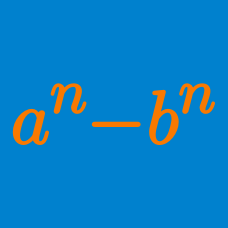Algebra

# Factorization of Quadratics

If $x-y-5=0$, then the polynomial $3x^2+xy-y^2-25x-y+50$ can be simplified as $ay(y+b)$. What is $a+b$?

Given that \begin{aligned} & x = -a+b+c, \\ & y = a-b+c, \\ & z = a+b-c ,\\ & a^2+b^2+c^2 =20, \end{aligned} find the value of $x^2+y^2+z^2+xy+yz+zx$.

Suppose that $a$ is an integer such that $x^2+2xy-80y^2+ax+18y+81$ can be factorized as the product of linear expressions in $x$ and $y$. What is the value of $a$?

$a, b$ and $c$ are integers such that $(x-a)(x-20) + 1 = (x+b)(x+c) .$ What is the sum of all possible values of $a$?

Suppose $a$, $b$ and $c$ are real numbers such that $a+b+c=5.$ If $x=a-2b+3c, y=b-2c+3a, z=c-2a+3b,$ what is the value of $(x^2+2xy+4)+(y^2+2yz+4)+(z^2+2zx+4)?$

×